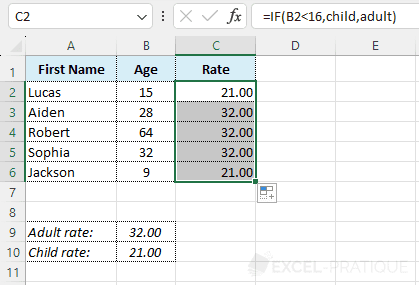# Excel Function: IF

The Excel IF function allows the display of different data depending on the result of a test.

Usage:

`=IF(condition, value_if_true, value_if_false)`

## Usage example

In the "Rate" column, the amount should be 21 for children (under 16 years) and 32 for others.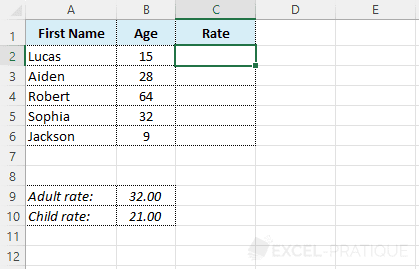To simplify the reading of the formula, you can start by renaming the cells B9 and B10.

To do this, click on B9 (see image), write "adult" and press Enter: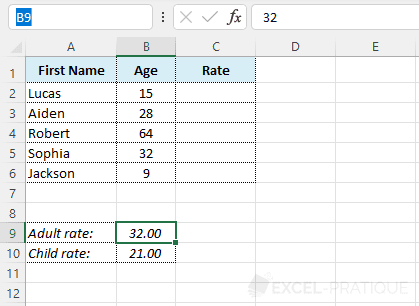Do the same with cell B10 and write "child" in it: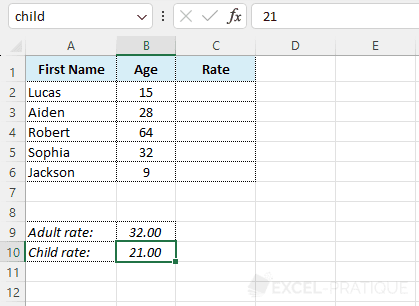Now select cell C2, click on "Insert Function" and select the IF function: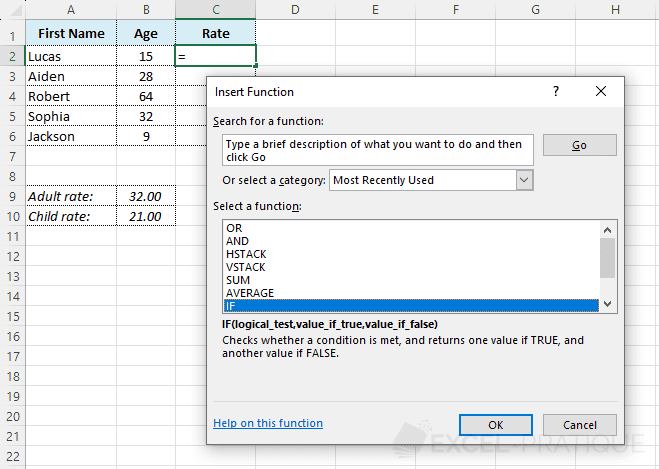In the Logical_test field, the test `B2<16` will check if the age is less than 16 years.

If this turns out to be true, the function should display the child rate and otherwise the adult rate:

``=IF(B2<16,child,adult)``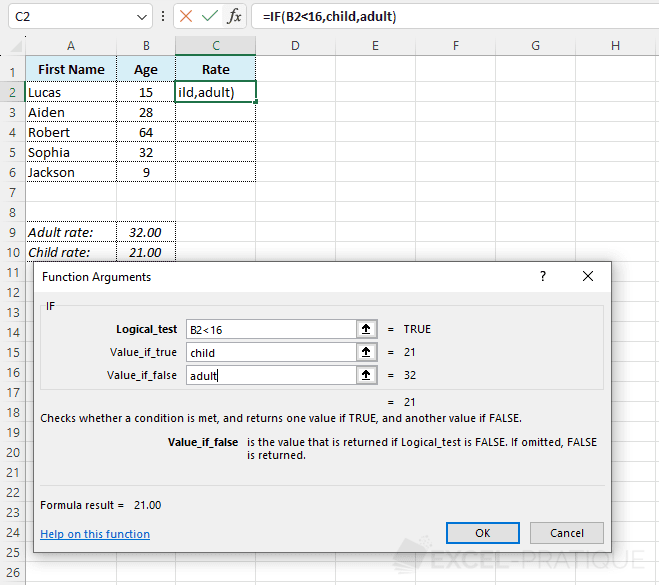Click on OK.

The result is displayed.

Then all you have to do is drag the formula down: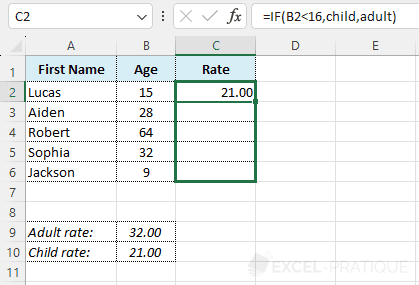To get: